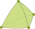####Shinohara : 数学講究 [Public]

By Shinohara Takuma  ( )

By kaoru  ( )

By kaoru  ( )

####FMFEM : Vibrations of Plates [Public]

By kaoru  ( )

Simulation of Chladni's figures, and Python code for Fujino-Morley FEM.

By kaoru  ( )

たわみを文字式のまま解く

By kaoru  ( )

####Hypercircle : Steklov Eigenvalue [Public]

By liqin  ( )

By using the hypercircle method, this project describes how to obtain the guareenteed lower eigenvalue bound of Steklov operator with FEM.

####EigenfunctionEstimation4FEM : Eigenfunction Estimation based on FEM solutions (Clone from xfliu/EigenfunctionEstimation4FEM) [Public]

By mahdipc  ( )

This project demonstrate the eigenfunction estimation of Laplacian using finite element method.

####Guaranteed_local_error_estimation : Local error estimation in JCAM [Public]

By nakano  ( )

This project provides a numerical example shown in the following article: https://www.sciencedirect.com/science/article/pii/S0377042723000055

####Guaranteed_error_estimation_for_modified_Helmholtz_eq : Guaranteed_error_estimation_for_modified_Helmholtz_eq [Public]

By nakano  ( )

This project provides computational examples related to guaranteed error estimation for the modified Helmholtz equations. The computation method used in this project is based on the approach presented in the research paper available at arxiv.org:2001.09820, as well as the project available at https://ganjin.online/liqin/Hypercircle.

By ryoki  ( )

####DirichletSimplicty : the 2nd and 3rd eigenvalues on triangles [Public]

By ryoki  ( )

Computer-assisted proof for the simplicity of the 2nd and 3rd dirichlet eigenvalues on triangular domains

####ShapeDerivativeEstimation : Rigorous shape derivative of eigenvalues estimation [Public]

By ryoki  ( )

This project demonstrates an implementation of the algorithms proposed in "Shape optimization for the Laplacian eigenvalue over triangles and its application to interpolation error constant estimation".

####InterpolationErrorEstimate : Interpolation error estimate [Public]

By shirley  ( )

Demonstration of the method of estimating the upper bound of the constant for the maximum norm of the Lagrange interpolation error.

####Pointwise : Pointwise (Clone from nakano/Pointwise) [Public]

By shirley  ( )

Pointwise error estimation based on the Fujita method for the Poisson BVP.

By vlabshs  ( )

####NS.SolutionVerification : Solution verification for the Navier-Stokes equation [Public]

By xfliu  ( )

Demonstration of the solution verification for the Naiver-Stokes equation over 3D domains.

####Hypercircle : The hypercircle method in the error estimation for FEM [Public]

By xfliu  ( )

This project describes how to obtain explicit a priori error estimation for FEM solution by using the hypercircle method.

####StokesEigProblem [Public]

By xfliu  ( )

The eigenvalue estimation for Stokes operator.

####vfem : Verified Finite Element Method Library [Public]

By xfliu  ( )

The Verified Finite Element Method (VFEM) library

####StokesEquation [Public]

By xfliu  ( )

The a priori error estimation for the FEM solution to Stokes equation over 3D domain.

####ProjectConstantCR [Public]

By xfliu  ( )

The projection error constant estimation for Crouzeix-Raviart FEM.

####Matrix4StokesEquation : Matrix generation in solving the Stokes equation by FEM [Public]

By xfliu  ( )

In solving the Stokes equation, one has to consider the solution of linear system under a linear constraint condition. Here, the code is prepared for the matrix generation.

####OctaveInterval : Example of Interval package in Octave [Public]

By xfliu  ( )

The project show several examples about interval computing in Octave.

####EigenVecEstimation [Public]

By xfliu  ( )

This project shows a demo of the algorithm of the a posteriori error estimation for eigenvector of eigenvalue problems, such as, the matrix eigenvalue problem, the Laplacian eigenvalue problem.

####EigenfunctionEstimation4FEM : Eigenfunction Estimation based on FEM solutions [Public]

By xfliu  ( )

This project demonstrate the eigenfunction estimation of Laplacian using finite element method.

####Book-GCEVP : Book: Guaranteed computation methods for eigenvalue problems of differential operators [Public]

By xfliu  ( )

The codes for numerical examples in the book: Guaranteed computation methods for eigenvalue problems of differential operators.

####LG-Method : Lehmann-Goerisch Method for high-precision eigenvalue bounds [Public]

By xfliu  ( )

The project demonstrates the high-precision eigenvalue bounds via Lehmann-Goerisch method

####EigenvalueBound : Eigenvalue bounds for Laplacian [Public]

By xfliu  ( )

The explicit lower bound and upper bound for the Laplace differential operator are obtained by using the conforming and non-conforming finite element method.